CiteScore 2.2
More on impact ›

# Frontiers in Built Environment## Original Research ARTICLE

Front. Built Environ., 02 June 2020 | https://doi.org/10.3389/fbuil.2020.00075

# Optimal Design of Block Quay Walls

• 1Structural Mechanics Section, Department of Civil Engineering, KU Leuven, Leuven, Belgium
• 2Engineering Department, BESIX, Brussels, Belgium
• 3Architectural Engineering Section, Department of Architecture, KU Leuven, Leuven, Belgium

Block walls, consisting of stacked unreinforced prefabricated concrete blocks, are commonly used for the construction of quay walls in the presence of rocky subgrades. A traditional design of block quay walls is based on manual design iterations, envisaging sufficient safety against ultimate limit states (ULS) such as sliding, overturning or loss of bearing capacity of the foundation soil. In addition, the designer should consider stability during the different construction stages of the block wall, referred to as construction constraints. This design process can be laborious, while the resulting designs comprise a large volume of concrete. In order to optimize block quay walls, we developed an automated design procedure in the framework of gradient-based optimization, accounting for the various ULS and construction constraints encountered in engineering practice. The design checks for a block quay wall are first explained in detail. This includes global ULS requirements that apply to the block wall as a whole, and internal ULS requirements to consider sliding and overturning of separate blocks. During all construction stages, the block wall has to be stable, which imposes additional construction constraints. Block walls consisting of rectangular blocks and chamfered blocks are optimized. The resulting designs obtained with the automated design procedure satisfy all design requirements, and have a realistic layout. Furthermore, the influence of the different construction stages is studied, demonstrating the practicality of the proposed automated design procedure.

## 1. Introduction

Block walls are gravity retaining walls that consist of unreinforced prefabricated concrete blocks. While block walls use a lot of material, they are commonly used as a retaining wall in the case of rocky subgrades that are characterized by a large bearing capacity, and where soil penetrating constructions such as combi-walls, sheet piling, or slurry walls are difficult or impossible to install (de Gijt and Broeken, 2013). Block quay walls reach typical retaining heights of up to 30 m and can be both constructed in dry conditions, or by placing blocks under water. The blocks are commonly prefabricated near the construction site, and stacked using construction cranes. Block quay walls are characterized by permeable joints, providing drainage that results in little or no water pressure differences over the wall.

A traditional design of block quay walls is based on manual iterations, governed by ultimate limit states (ULS) that require sufficient safety against failure mechanisms such as sliding, overturning or loss of bearing capacity of the foundation soil (de Gijt and Broeken, 2013). This traditional design approach, with manual iterations based on engineering judgement, is laborious and time consuming. Therefore, the question arises whether numerical optimization techniques could yield similar or better, more economic designs, at the same time reduce engineering costs.

Numerical design optimization has already been applied to a variety of geotechnical applications, including the design of piled foundations (Chan et al., 2009), underground excavations (Ren et al., 2014), and rock bolt design (Nguyen et al., 2015), mostly based on genetic algorithms and simplified design methods. For example, for cantilever retaining walls, Camp and Akin (2012) and Kaveh et al. (2011) employ a Big Bang-Big Crunch optimization algorithm, Khajehzadeh et al. (2013), Moayyeri et al. (2019), and Talatahari et al. (2012) use a gravitational search in the context of particle swarm optimization, whereas Gandomi et al. (2016) and Nandha Kumar and Suribabu (2017) apply evolutionary algorithms. While such genetic algorithms are easy to understand and implement, they can only cope with a limited number of design variables, such as the geometry of a cantilever wall that is described by a limited number of parameters. Furthermore, genetic algorithms require many iterations to converge. As the number of design variables increases, a gradient-based optimization algorithm becomes more attractive, while requiring careful treatment of the objective function and constraints. For the particular case of block quay walls, such a numerical optimization has been applied by Shafieefar and Mirjalili (2013) and Shafieefar and Mirjalili (2014), using a gradient-based sequential quadratic programming algorithm. This algorithm is particularly suited to cope with the large design freedom, varying the number, size and position of the blocks.

The typical loads for block quay walls include the weight of the blocks, the lateral earth pressures on the blocks, water pressures, surcharge loading, and loading by mooring ships. As design requirements, sufficient safety against sliding, overturning or loss of bearing capacity of the foundation soil are imposed. In addition, seismic loads are to be considered in seismic prone areas (Richards Jr. and Elms, 1979). A classical quasi-static seismic design commonly used in practice is based on the use of seismic earth pressure coefficients obtained from a Mononobe-Okabe analysis. Such a quasi-static analysis represents a strong simplification, and a lot of research is devoted to provide improvements, such as Newmark's sliding block method (Li et al., 2010; Caltabiano et al., 2012; Pain et al., 2017) or the use of dedicated models to account for the behavior of the interfaces between the blocks under complex loading scenarios, such as torsional effects (Casapulla and Maione, 2018; Casapulla et al., 2019). In the present paper, however, seismic loads are not considered.

Apart from these essential ULS stability requirements, the design of block walls also includes considerations on constructability: the block wall should be stable at every stage of the construction of the wall, and engineering a construction sequence forms an integral part of the design. Block walls are typically gradually backfilled a couple of times during construction for logistical reasons. The number of backfill operations and the corresponding stacking sequence hence provides additional design freedom, where the stability of the freestanding stack of blocks is considered as an additional constructability constraint. Other constructability constraints include e.g. a maximum block weight, governed by the cost and availability of construction cranes.

This paper develops a gradient-based shape optimization algorithm for block walls, aiming at reducing the material use while accounting for realistic boundary conditions, load cases, and construction constraints. The width, height, and position of the blocks are adjusted in order to obtain an optimal layout. Accounting for ULS envisages sufficient safety against failure mechanisms such as sliding, overturning, or loss of bearing capacity of the foundation soil. Additional construction constraints include the maximum weight of the blocks and the stability of the block wall during construction. This constructability constrained optimization represents a first step toward a holistic design approach to quay walls (de Gijt, 2015), aiming at an optimal technical and economical design, and considering the total lifecycle costs that include the costs of engineering, construction, maintenance, demolition and environmental impacts.

This paper is organized as follows. In section 2, the design requirements are outlined by means of an example block wall, for which the different elements of the analysis are explained in detail. In section 3, the shape optimization problem is defined for a wall consisting of rectangular blocks, where a two-block wall allows to visualize the optimization process. Furthermore, the results of an optimization of a realistic wall with more blocks are discussed. Since chamfered blocks are commonly used in practice and allow for more design freedom, section 4 extends the optimization problem to such chamfered blocks, and the optimization results are compared with the case of rectangular blocks. Section 5 summarizes the main findings of the paper.

## 2. Block Wall Design

### 2.1. Problem Outline

In order to demonstrate the design principles of a block wall, a simple layout with four rectangular blocks is considered, as shown on Figure 1. The base of the wall is located at z = −6m, and every block i = 1, …, nblock, nblock= 4 has a height ti = 2m so that the total wall height Htot = 8m. Furthermore, the water level wl = −0.5m on the left side is lower than the water level wr = 0m on the right side, resulting in an unfavorable water pressure on the wall. In the following sections, the material properties of the blocks, the backfill, and the foundation soil are listed. The forces acting on the structure are subsequently discussed, such as variable loads, effective soil pressures, and water pressures. This allows to check ULS requirements, including sliding failure, overturning, and loss of bearing capacity of the soil. Global ULS requirements apply for the block wall as a whole, whereas internal ULS requirements consider sliding and overturning for each block separately.

FIGURE 1

#### 2.1.1. Material Properties

The concrete blocks are considered to be rigid and have a unit weight γc = 24 kN/m3. The blocks have a compressive strength fcu = 45 MPa, which limits the stresses at the interface between the blocks. The foundation soil is characterized by an internal friction angle ϕ = 28°, a cohesion c = 83kPa, and dry and saturated unit weights ${\gamma }_{\text{dry}}=20\text{kN}/{\text{m}}^{3}$ and ${\gamma }_{\text{sat}}=22\text{kN}/{\text{m}}^{3}$, respectively. These soil properties are representative for rocky soils. The backfill is characterized by an internal friction angle ϕ = 40°, a zero cohesion c = 0kPa, and dry and wet unit weights ${\gamma }_{\text{dry}}=20\text{kN}/{\text{m}}^{3}$ and ${\gamma }_{\text{sat}}=22\text{kN}/{\text{m}}^{3}$, respectively. Furthermore, the strength of the soil-block interface is characterized by an interface friction angle δ = 2/3ϕ.

The variable loads include the point loads Qx and Qz, distributed vertical loads qz on top of the wall, and surcharge loading q0 next to the wall (Figure 1). Four load cases (LCs) are considered, denoted as LC1 to LC4, as listed in Table 1. Sliding failure of the wall dominates LC2, in absence of the vertically distributed load and vertical point load on top of the wall. Overturning of the wall governs LC4, whereas the loss of load bearing capacity of the soil is dominant for LC3, with all loads considered. A negative Qx and q0 are always unfavorable, and are considered in every LC.

TABLE 1

#### 2.1.3. Soil Pressures

The backfill generates soil stresses that represent the predominant loading on the block wall. Since the block wall has a denticulated back, the calculation of soil pressures is more complex than for straight retaining walls such as diaphragm walls. The soil pressures on block walls are computed by considering a vertical fictitious back plane (Figure 1), as described in the British Standard (BSi, 2015). In a manual design calculation, the fictitious back plane is determined on the basis of engineering judgement, roughly following the back of the block wall. In order to compute soil stresses in a gradient-based optimization scheme, an automated calculation is required. Therefore, the convex hull of the ensemble of blocks is considered as the fictitious back plane, since its sound mathematical definition allows for an algorithmic treatment. The fictitious back planes for the example block wall, computed from the convex hull, are shown in Figure 2. A different fictitious back plane is considered for the equilibrium of each block.

FIGURE 2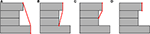Figure 2. Convex hull as the fictitious plane for the equilibrium of (A) block 1, (B) block 2, (C) block 3, and (D) block 4.

On the fictitious back plane, the coefficient of active soil pressure is computed using the method of Müller-Breslau (1906), assuming a straight slip surface of the failure wedge behind the wall:

where α is the slope of the fictitious back plane, β the slope of the ground surface (considered zero in this paper), and δ is the wall friction. The active soil pressure coefficients are listed for each block in Table 2.

TABLE 2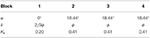Table 2. Active soil pressure coefficient Ka at each block of the example wall for global equilibrium, including the angle α of the fictitious back plane and the angle of friction δ of each block.

While the wall can have a small embedment depth in which (stabilizing) passive soil pressures could be generated, these passive soil pressures are neglected since the soil near the front of the block wall is often eroded or stirred as a result of the construction work.

#### 2.1.4. Weight of the Enclosed Soil

Apart from the weight of the blocks, the weight of the soil above protruding blocks (Figure 3) provides a stabilizing effect on the sliding and overturning failure mechanisms. The net weight of this enclosed soil is accounted for in order to avoid overconservative designs. Furthermore, buoyancy results in a weight difference of the soil above and below the water table.

FIGURE 3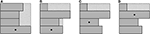Figure 3. Enclosed soil (hatched) for a number of wall layouts, where the dot indicates the block for which equilibrium is considered.

#### 2.1.5. Water Pressures

The water pressure acting on the example wall is shown in Figure 4, where a distinction is made between the water pressure acting on the block wall as a whole (for global equilibrium) and the water pressure acting on individual blocks (for internal equilibrium). The water pressure is linearly interpolated along the bottom and top surfaces, accounting for water flow through the permeable joints.

FIGURE 4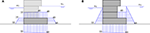Figure 4. Resulting water pressure (A) on the entire wall, when considering global equilibium, and (B) on block 1 when considering internal equilibrium.

### 2.2. Global ULS Requirements

The global ULS requirements include sliding and overturning of the block wall, as well as loss of bearing capacity of the soil. The definition of the corresponding safety factors for each of these failure mechanisms is discussed next.

The global safety factor of the sliding failure mechanism is defined as:

where Vres and Hres are the resulting vertical and horizontal forces, respectively, and fcg = 0.6 is the Coulomb friction coefficient between the bottom block and the foundation.

The global safety factor for overturning:

accounts for the resulting stabilizing moments Mstab and the resulting destabilizing moments Mdestab around the toe of the block wall.

The foundation has a width B, corresponding to the width of the first block. The resulting vertical force Vres and horizontal force Hres acting on the foundation are calculated from the sliding equilibrium. In order to compute the eccentricity of the force on the foundation, the moment relative to the center of the foundation Mcenter is required. The moment around the toe of the wall ${M}^{\prime }={M}_{\mathrm{\text{stab}}}$ + Mdestab. The moment around the center of the foundation Mcenter is computed as:

The eccentricity e of the load is computed as:

The effective foundation width equals B′ = B − 2e and the pressure on the foundation qeff is calculated as:

The bearing capacity qult is computed using Terzaghi's equation (Tomlinson and Boorman, 2001):

where Nc, Nq, and Nγ are bearing capacity coefficients, represented by non-linear functions of the angle of internal friction ϕ. In the present paper, the equations of Meyerhof are applied (Tomlinson and Boorman, 2001):

The parameters a and b are 0.1054 and 9.6, respectively, for a rough foundation. The depth factors dc, dq and dγ are equal to 1 because a surface foundation is considered. The form factors sc, sq, and sγ are also equal to 1 for the plane strain case at hand. The slope factors ic, iq, and iγ take into account the angle at which the resulting loads occur, and are calculated as:

where q is equal to the surcharge next to the foundation. The effective width B′, the horizontal and vertical resulting forces Hres and Vres are already known. ϕ and c correspond to the internal friction angle and the cohesion of the foundation soil and the effective bulk weight equals ${\gamma }^{\prime }={\gamma }_{\mathrm{\text{sat}}}-{\gamma }_{\mathrm{\text{w}}}$ below the groundwater level and ${\gamma }^{\prime }={\gamma }_{\mathrm{\text{dry}}}-{\gamma }_{\mathrm{\text{w}}}$ above the groundwater level. Applying Terzaghi's formula to the example block wall, the second term in Equation (7) is omitted because there is no effective vertical stress q′ on the foundation.

The global safety factor for the bearing capacity of the soil is defined as follows:

The safety factors for the example block wall for all four load cases are shown in Table 3. It should be noted that sliding is the most critical failure mechanism. For sliding, LC2 is critical since there are no vertical loads on top of the quay wall. Overturning is critical for LC2 and LC4. The bearing capacity controls LC3, since all vertical loads are present. The vertical point load at the top of the wall has a major influence on the foundation pressure qeff for two reasons: (a) the eccentricity of the load considerably reduces the effective width Beff, and (b) the additional vertical forces cause higher stresses on the foundation. The increase in foundation pressure qeff is the main reason for the reduction of the safety factor for bearing capacity rather than the decreasing slope factors ic, iq, and iγ, as a consequence of the large eccentricity of the external load.

TABLE 3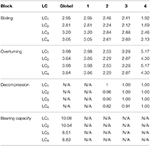Table 3. Factors of safety for the global and internal ULS requirements for the example block wall.

### 2.3. Internal ULS Requirements

The eccentricity of inter-block forces must be restricted so that a minimum contact area is subjected to pressure, avoiding decompression (loss of contact stresses) on one side of the contact surface, at the same time preventing excessive compressive stresses on the (small) remaining contact area. The compression ratio Ccompression,k is the ratio between the compressed part ${A}_{k}^{\prime }$ and the total contact surface Ak between two blocks:

The index k refers to the upper block and varies from 2 to nblock. In addition, the maximum contact stress σmax,k between the blocks is also restricted. The compression ratio and the maximum contact stress depend on the eccentricity ek of the vertical force Vk on the contact surface k. If ek/Bk < 1/6, the vertical contact force is located in the central core (middle third) of the contact surface, and decompression does not occur, i.e., Ccompression,k = 1. Correspondingly, the maximum contact stress equals:

If ek/Bk > 1/6, the vertical force is located outside the central core, resulting in decompression. The compression ratio is then calculated as:

in which case the maximum contact stress σmax,k equals:

where Bk is the width of the contact surface. The eccentricity ek of the vertical force is calculated as ${e}_{k}=\frac{{M}_{\mathrm{\text{center}},k}}{{V}_{k}}$. The maximum contact stress of σmax,k is compared to the ultimate compressive strength of the blocks fcu by means of the ratio of Fσmax,k:

The safety factors for the internal ULS requirements are shown in Table 3 for each load combination. It should be noted that the safety factors for sliding and overturning of block 1 are equal to those of the global equilibrium (Table 3). Furthermore, the upper block 4 is the most sensitive to sliding due to a large horizontal point force on the top of the wall. Therefore, in practice, the upper block is typically casted continuously in-situ as a capping beam in order to distribute the forces over larger portions of the block wall. The overturning failure mechanism is critical for block 2. Decompression does not occur and the maximum stresses are limited. Therefore, concrete C35/45 with a compressive strength fcu = 45 MPa is more than sufficient to resist the resulting stresses.

### 2.4. Constraints on Protrusion

Blocks that excessively protrude above the underlying block are prone to break under their own weight or may overturn during construction. Therefore, two conditions are imposed for all but the first block (k = 2, …, nblock). The first condition prevents breakage: blocks may not protrude over a distance more than their height. This rule is also referred to as the 45° rule because cracks in concrete are considered at an angle of 45°. The condition can be expressed with a safety factor SFprotrusion1,k:

where tk is the thickness and Uk is the protrusion length of the considered block. The safety factor is calculated for protrusions at the front as well as at the back of the quay wall. For the example block wall under consideration, only protrusion at the back is relevant.

The second condition prevents the blocks from overturning during construction. The center of gravity of block k is enforced to be located within the contact surface with the supporting block k − 1. The corresponding safety factor SFprotrusion2,k is defined as:

where lk is the width of the supporting and Lk is the width of the block.

### 2.5. Construction Constraints

The above safety factors guarantee sufficient safety with respect to ULS failure mechanisms. However, the global and internal ULS requirements assume that the block wall is supported by the backfill. For logistical and financial reasons, the block wall is only backfilled once or a couple of times during construction. As a result, the stability of the freestanding stack of blocks has to be guaranteed during the construction of the wall. If a number of blocks is stacked, overturning of the stack should be avoided. The most unfavorable situation occurs when the backfill is completed after stacking of the entire block wall.

In order to account for constructability, safety factors are defined to allow to stack a number of blocks in each building stage without the need for gradual backfilling. If two blocks have been stacked, the center of gravity of the stack must be within the contact surface with the lower block, if three blocks have been stacked, the center of gravity of the stack must meet these conditions, and so on. In the following, we consider nstage construction stages where nblocki represents the number of blocks placed in stage i = 1…nstage, and consider stability of the freestanding stacks of blocks. The safety factors SFconstructability,m,n for the constructability of stage m are expressed as follows:

where zk,n is the center of gravity of the group of blocks of the considered check n. Lfic,k,n is the fictitious length of the group of blocks of which the center of gravity zk,n is calculated. This fictitious length is obtained by dividing the area of the group of blocks under consideration by the total height of the group. The index n varies from 1 to (nBlockinStage,m × (nBlockinStage,m − 1)). The checks are performed for overturning to both sides. For the 4-block example, this corresponds to the six safety factors listed in Table 4.

TABLE 4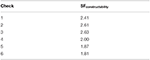Table 4. Safety factors for the constructability of the example block wall in a single stage.

## 3. Optimization Using Rectangular Blocks

### 3.1. Optimization Problem

The geometry of a block wall with rectangular blocks is uniquely defined by the number of blocks nblock in the wall, the width Li and height ti, and the x-coordinate of the bottom left corner of each block xi. Therefore, the complete quay wall layout is described by the vector of design variables x:

where x1 and xn are fixed at x1 = 0 and xn = 0. In addition, the height of the highest block ${t}_{n}={H}_{\mathrm{\text{tot}}}-\sum _{k=1}^{n-1}{t}_{k}$ is not a design variable since the total height Htot of the wall is fixed a priori. As a result, the number of design variables equals nvar = 3nBlocks − 3.

The total cost of a block wall depends on many factors including logistic considerations, location of the construction site, and availability of equipment and is therefore difficult to objectively determine. Therefore, the total volume of concrete is considered as the objective function f0 to be minimized.

Most of the constraints to which the design has to comply correspond to the ULS safety of the block wall. The safety factors SFsliding,k, SFoverturning,k, and SFbearing capacity are restricted in order to provide sufficient stability of the block wall, both considering global and local equilibrium. Furthermore, the compression ratio Ccompression,k is constrained, limiting the stress Fσmax,k. In addition, cantilever equilibrium correspond to the factors SFprotrusion1,k and SFprotrusion2,k and the construction constraints in correspondence to the safety factor SFconstructability,m,n should apply.

Furthermore, a weight limit Mmax per block is imposed. This limit is case specific and corresponds to the maximum capacity of the cranes available at the construction site. Furthermore, the contact area between the different blocks must have a minimum width Bmin in order to prevent local stress concentrations and failure. The minimum width of the contact surface is a constraint, in addition to the sliding constraint: sliding is only verified based on the ratio of forces and does not take into account the size of the contact surface. Finally, the relative weight difference between blocks is limited, avoiding large variations in block weight and reducing overall construction costs.

The various constraints are collected in the vector f:

where

### 3.2. Optimization Algorithm

An appropriate algorithm is selected for the solution of the constrained optimization problem. Since the number of blocks in a block wall is rarely larger than about 15, and the forward calculation takes very little computation time, the sensitivities can easily be determined numerically by means of finite differences. Therefore, a gradient-based optimization algorithm is preferred. In this paper, the active set method, as implemented in the Matlab function fmincon, is employed. The active set method turned out to be the most performant algorithm provided by the fmincon function. The active set method typically works well for highly constrained problems, as in the present case. The following convergence requirements have been considered: iterations are performed until a step tolerance $\Delta {f}_{0}=1{0}^{-8}$ of the objective function is reached. A design is considered feasible if the constraints max(f) is less than the assumed value 10−4, and the maximum number of iterations of the search algorithm is set to 70. The gradients of both the objective function as well as the constraints are computed using a finite difference approach.

### 3.3. Optimization of a Two-Block Wall

The optimization algorithm is first applied to a block wall consisting of 2 blocks, only considering the lengths L1 and L2 of the blocks as design variables. The objective function and the constraints can thus be visualized using a contour plot in (L1,L2)-space. This visual representation allows to check whether the search algorithm actually converges to the desired optimum. For various start values of the algorithm, the same optimum is found. In the optimum (L = {4.9615m, 6.6154m}T), all design requirements are met.

Figure 5 shows a contour plot of the objective function and all constraints. For sliding failure, LC2 is controlling the design. For the overturning failure mechanism, LC2 and LC4 are controlling. LC1 or LC4 control the loss of bearing capacity as well as the decompression, depending on the value of the design variables L1 and L2. This example demonstrates the importance of taking all load cases into account.

FIGURE 5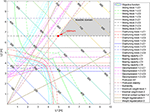Figure 5. Contour plot of the objective function for the 2-block wall as a function of the design variables L1 and L2. The constraints, the feasible domain, and the optimum are also indicated.

The constraints for sliding, overturning, and maximum weight of block 2 are linear or constant and only depend on L2. Similarly, the protrusion, construction, and weight regularization constraints are linear in L1 and L2. However, the constraints on sliding and overturning of block 1 depend on both L1 and L2 in non-linear way. This can be explained as follows: at a value of L1 = 7.7m for the sliding failure and L1 = 5 m for overturning, the weight of block 2 is not required for stabilizing block 1. As a result, the constraint does not depend on L2. However, if L1 becomes smaller, the constraints require an increasing length L2 of block 2 to provide sufficient safety against sliding and overturning of block 1.

The contour lines of the constraints on decompression and bearing capacity are non-linear, due to the different conditions in calculating those safety factors. It should be noted that L2 should not be less than 3.5m to comply with the decompression requirements. If the length is greater than L2, the length L1 must also be greater for the same reason. The bearing capacity requirement imposes a minimum length L1 = 5 m. From length L2 = 4.5m, an increasing L2 involves increasing L1 in order to resist the extra weight of block 2.

At the bisector of the contour plot in Figure 5, a discontinuity occurs in constraints of the (a) sliding failure of block 1, (b) tilting of block 1, (c) loss of bearing capacity of the wall and (d) decompression of the contact area between block 1 and block 2. These discontinuities occur when L1 = L2, and the friction angle on the fictitious back plane is replaced by an interface friction angle δ.

### 3.4. Optimization of a Wall With 12 Blocks

The algorithm is also used to optimize the design of a wall with 12 blocks and a retaining height of 30 m. The base level is located at z = −28 m. The soil properties, the water levels, the forces on the wall and at ground level, the load cases, the concrete strength and the friction coefficients are the same as for the example block wall.

The design variables are described in Equation (23), where the x-coordinates of the left corner of the lowest and highest block are fixed to 0. The thickness of the highest block is fixed in order to obtain a retaining height of 30 m. The resulting number of design variables is 33. To be realistic, the blocks are limited to a maximum weight of 80 tons. Since the blocks are typically 2 m long, the weight limit is set at 40 tons/m. For the lower block, however, the weight is increased to 80 tons/m in order to have a sufficient bearing capacity. The factor Fweight is equal to 0.5 and the minimum width of the contact surface between the blocks Bmin is fixed at 4 m. The design variables are box constrained: the block width varies between 1 and 15 m, the x-coordinate between 0 and 5 m and the heights of the blocks range from 0.5 to 4 m. The height of the lower block, however, is limited to a minimum of 2 m because that block must be sufficiently thick so it will not break and provide sufficient load-bearing capacity.

The outcome of the active-set optimization algorithm strongly depends on the initial values of the design variables. Different initial values can lead to convergence to (sub)optimal solutions. Therefore, series of 100 simulations are performed with random initial values and the five best designs are retained. If the total volume of the five best designs are very close to each other, it is assumed that the optimum has been found. The initial values are randomly selected but (1) must meet the box constraints, (2) must have a positive initial value for the height of the upper block and (3) the contact surface between blocks must have a minimal width of 1 m.

Six different building scenarios for the wall are considered. The first scenario simulates the design of a 12-block wall in a single stage. Scenario 2 to 6 simulate series in which construction takes place in 2 stages of 6 blocks, 3 stages of 4 blocks, 4 stages of 3 blocks, 6 stages of 2 blocks, and 12 stages of a single block, respectively. The optimization problem has 33 design variables and 531 constraints (at maximum, when nStage = nblock). Simulation times vary between 20 and 40 s on a regular PC. Since the optimization problem is non-convex, a series of 100 designs is obtained from random starting values, and the best designs are retained. Computing 100 designs takes 1 h. The total volume and wall layout of the best designs of the hundred simulations is shown in Figure 6.

FIGURE 6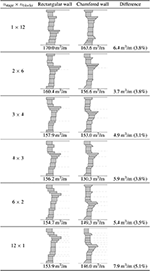Figure 6. Optimal designs for the rectangular and chamfered block wall, including the resulting wall volume. The gradual backfill stages are indicated with dashed lines.

Figure 6 also shows how the volume evolves with the number of construction stages, ranging from 170.0 m3/m for a single stage to 153.9 m3/m for construction in 12 stages. Additional construction stages allow to further reduce the volume of the design. The results can be used to perform a cost-benefit analysis to determine an optimal number of construction stages: if replenishment of the backfill is relatively inexpensive, then a choice for 3, 4, or 6 construction stages is an excellent choice. If the cost of additional construction stages is larger than the reduced material cost, then building in 1 or 2 stages is recommended. A maximum of approximately 15.2 m3/m of concrete can be saved by building in 6 stages compared to building in 1 stage. The difference between building in 3 or 6 stages is small.

In the optimized designs, as shown in Figure 6, a relief platform can be observed at the level of blocks 8, 9 or 10. The relief platform is located at a position low enough to allow the stabilizing action of the superimposed mass of soil and high enough so that it is beneficial for the internal equilibrium of 8 to 10 of the 12 blocks. In the case of series 1, the platform is at a lower position near block 5. The reason for this is that with a higher platform, as in series 4, the structure is not stable during the construction of the wall in 1 stage. Therefore, the platform is located at a lower position. The blocks above that location are almost all large blocks that keep themselves in balance and provide the counterweight to balance the earth pressures. Series 2 is of an intermediate form, with a platform at the height of block 8. At the front of the quay wall, an arch shape can be seen in each case. The arch at the front can be explained by the effect of force around the toe of the wall: the more the blocks are located to the rear, the greater the lever arm of their weight and therefore the greater the stabilizing moment that prevents tilting.

## 4. Optimization Using Chamfered Blocks

### 4.1. Optimization Problem

In practice, the corners of the rectangular blocks are commonly chamfered, so that a smooth face is obtained. This section investigates to what extent the use of chamfered blocks can further optimize the design. Chamfered blocks are characterized by the lengths of the blocks L, the x-coordinates of the bottom left corner of the blocks x and the heights of the blocks t. The chamfers, considered at an angle of 45° as shown in Figure 7, are 4 additional parameters, where the height of the chamfers is expressed as a percentage (d1i to d4i) of the block height. The vectors d1, d2, d3, and d4 are added to the set of design variables:

FIGURE 7

The chamfers d1 and d4 of the lowest block and d2 and d3 of the highest block are not considered, in accordance to actual engineering practice. The number of design variables nvar increases to 7 × nblock − 7 with respect to that of the rectangular block wall.

Additional constraints f12 to f20 are considered for the chamfered case:

where:

The constraints f12 and f13 impose that the two lower and the two upper chamfers of a block do not exceed the block length. The constraint f14 ensures that the width of the top of the quay wall always remains greater than the minimum width Bmin, considered 4 m in this paper. The conditional constraints f15 to f18 have been introduced to avoid chamfers as shown in Figure 8A, since such a situation is never considered in practice where a layout as shown in Figure 8B is commonly preferred. Figure 8A shows that both parameters UH1 and UH2, and the bevel d12 of the upper block are positive and therefore the limitation is not met. For all previous conditional constraints, a term Fcond has been added to improve the convergence of the algorithm. The term Fcond takes a small value and allows the constraints to be violated in a controlled way, where the tolerance is determined by trial-and-error.

FIGURE 8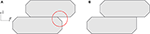Figure 8. (A) A chamfer underneath a jump is (B) avoided by imposing constraints f15 and f17.

Constraint f19 imposes that either bevel d1 or bevel d2 of a particular block must be equal to zero and limitation f20 that either bevel d3k or bevel d4k of a particular block k must be equal to zero. This corresponds to a bevel either at the top or at the bottom corner. Correspondingly, the blocks can only take a trapezoidal shape, avoiding octagonal shapes.

### 4.2. Optimization of a Wall With 12 Blocks

In this section, a block wall consisting of 12 chamfered blocks is optimized. Apart from the chamfering, all input parameters and algorithmic parameters correspond to the 12 rectangular block case. Box constraints are also applied, where the length of the blocks varies between 1 and 15 m, the x-coordinate varies between 0 and 5 m and the height of the blocks varies from 0.5 to 4 m. The thickness of the lower block is limited to at least 2 m. The parameters relating to the chamfers may vary from 0 to a maximum of 70% in order to avoid sharp, fragile corners.

A series of 50 designs is simulated using random start values and the best designs are retained. The initial values meet the same conditions as specified in section 3. In addition, the initial values must meet the following condition to prevent chamfers from exceeding the length of that side:

Per series, 50 simulations have been conducted. Each series contains different construction constraints, i.e., building the wall in 2 stages of 6 blocks, 3 stages of 4 blocks, 4 stages of 3 blocks, 6 stages of 2 blocks, and 12 stages of a single block, respectively. The factor Fcond has been determined by trial-and-error to 0.025 for series 1 and series 3, and to 0.010 for series 2. The time to optimize the design is about 2 min, which is significantly longer than that for rectangular block walls. The results of different series are summarized in Figure 6.

The resulting designs are smooth at the front and the back. In each design there is a platform, which has also been observed in the design of block walls from rectangular blocks, that exploits the stabilizing effect of the soil. The resulting designs herein obtained are similar to the block walls that are being designed in practice.

The volume of the block walls does not differ much with respect to the number of construction stages nStage. Building in 2 stages is the most advantageous in terms of volume. The additional constraints, ensure that the construction constraints are only active to a limited extent and therefore have little influence on the total quay wall volume. The construction constraints therefore are less important in the general shape of the wall, in contrast to what was observed for the rectangular block walls.

## 5. Conclusion

In this paper, an automated design procedure for block quay walls taking into account construction constraints has been proposed in the framework of gradient-based optimization. Block walls consisting of rectangular or chamfered blocks have been considered, accounting for the various ULS and construction constraints encountered in engineering practice, aiming at minimal material use while maintaining a target factor of safety.

The design checks for a block quay wall have been explained in detail. This includes global ULS requirements that apply to the block wall as a whole, and internal ULS requirements that consider sliding and overturning of separate blocks. During construction, the stability of the block wall has to be guaranteed during all construction stages, which imposes additional construction constraints.

Block walls consisting of rectangular blocks and chamfered blocks have been optimized. The resulting designs obtained with the automated design procedure satisfy all design requirements, and have a realistic layout. The importance of the construction constraints has been demonstrated, as well as the corresponding additional volume of concrete in the wall. A number of typologies are identified, which depend on the construction constraints. In addition, the optimization of a block wall with chamfered blocks, as used in practice, results in very realistic designs, and allows for a fully automated design and optimization of the block walls. As such, the use of the proposed automated design procedure allows for a strong reduction of the engineering time, typically spent to manually optimize the design.

## Data Availability Statement

The datasets generated for this study are available on request to the corresponding author.

## Author Contributions

LL performed the implementation and analysis of the cases, where SF, MS, and HV were involved in the planning and supervision of the work. SF and LL prepared a first draft of the manuscript. All authors aided in interpreting the results and worked on the manuscript, discussed the results and commented on the manuscript.

## Conflict of Interest

The authors declare that the research was conducted in the absence of any commercial or financial relationships that could be construed as a potential conflict of interest.

## References

BSi (2015). Code of Practice for Foundations. BS 8004:2015.

Caltabiano, S., Cascone, E., and Maugeri, M. (2012). Static and seismic limit equilibrium analysis of sliding retaining walls under different surcharge conditions. Soil Dyn. Earthq. Eng. 37, 38–55. doi: 10.1016/j.soildyn.2012.01.015

Camp, C. V., and Akin, A. (2012). Design of retaining walls using big bang-big crunch optimization. J. Struct. Eng. 138, 438–448. doi: 10.1061/(ASCE)ST.1943-541X.0000461

Casapulla, C., and Maione, A. (2018). Modelling the dry-contact interface of rigid blocks under torsion and combined loadings: concavity vs. convexity formulation. Int. J. NonLinear Mech. 99, 86–96. doi: 10.1016/j.ijnonlinmec.2017.11.002

Casapulla, C., Panto, B., and Calio, I. (2019). “A new 3D-adaptive discrete interface for modeling the torsion behavior of masonry contact joints,” in Proceedings of the Int. Conf. on Computational Methods in Structural Dynamics and Earthquake Engineering (COMPDYN 2019) (Crete), 1–12. doi: 10.7712/120119.6949.19404

CrossRef Full Text

Chan, C., Zhang, L., and Ng, J. (2009). Optimization of pile groups using hybrid genetic algorithms. J. Geotech. Geoenviron. Eng. 135, 497–505. doi: 10.1061/(ASCE)1090-0241(2009)135:4(497)

de Gijt, J. (2015). The importance of hydraulic structures for society: quay walls and dikes in the Netherlands. Civil Eng. Dimens. 17, 179–186. doi: 10.9744/ced.17.3.179-186

de Gijt, J. G., and Broeken, M. (2013). Quay Walls. Boca Raton, FL: CRC Press.

Gandomi, A., Kashani, A., Roke, D., and Mousavi, M. (2016). Optimization of retaining wall design using evolutionary algorithms. Struct. Multidisc. Optim. 55, 809–825. doi: 10.1007/s00158-016-1521-3

Kaveh, A., Talatahari, S., and Sheikholeslami, R. (2011). “Optimum seismic design of gravity retaining walls using the heuristic big bang-big crunch algorithm,” in Proceedings of the Second International Conference on Soft Computing Technology in Civil, Structural and Environmental Engineering (Stirlingshire: Civil-Comp Press), Vol. 4, 10. doi: 10.4203/ccp.97.4

CrossRef Full Text

Khajehzadeh, M., Taha, M. R., and Eslami, M. (2013). Efficient gravitational search algorithm for optimum design of retaining walls. Struct. Eng. Mech. 45, 111–127. doi: 10.12989/sem.2013.45.1.111

Li, X., Wu, Y., and He, S. (2010). Seismic stability analysis of gravity retaining walls. Soil Dyn. Earthq. Eng. 30, 875–878. doi: 10.1016/j.soildyn.2010.04.005

Moayyeri, N., Gharehbaghi, S., and Plevris, V. (2019). Cost-based optimum design of reinforced concrete retaining walls considering different methods of bearing capacity computation. Mathematics 7:1232. doi: 10.3390/math7121232

Müller-Breslau, H. F. B. (1906). Erddruck auf Stuetzmauern. Kröner.

Nandha Kumar, V., and Suribabu, C. (2017). Optimal design of cantilever retaining wall using differential evolution algorithm. Int. J. Optim. Civil Eng. 7, 433–449. Available online at: https://pdfs.semanticscholar.org/b5e1/e2e1965d46331c035480baacbafc81953362.pdf

Nguyen, T., Ghabraie, K., Tran-Cong, T., and Fatahi, B. (2015). Improving rockbolt design in tunnels using topology optimization. Int. J. Geomech. 16:04015023. doi: 10.1061/(ASCE)GM.1943-5622.0000488

Pain, A., Choudhury, D., and Bhattacharyya, S. (2017). Seismic rotational stability of gravity retaining walls by modified pseudo-dynamic method. Soil Dyn. Earthq. Eng. 94, 244–253. doi: 10.1016/j.soildyn.2017.01.016

Ren, G., Zuo, Z., Xie, Y., and Smith, J. (2014). Underground excavation shape optimization considering material nonlinearities. Comput. Geotech. 58, 81–87. doi: 10.1016/j.compgeo.2014.02.003

Richards, R. Jr., and Elms, D. G. (1979). Seismic behavior of gravity retaining walls. J. Geotech. Geoenvironmental Engineering 105, 449–464.

Shafieefar, M., and Mirjalili, A. (2014). Cross section optimization of gravity type blockwork quay walls using sequential quadratic programming method. Int. J. Mech. 8, 234–241.

Shafieefar, M., and Mirjalili, M. (2013). “Optimization of block work quay walls cross section using SQP method,” in Proceedings of the 1st International Conference on Optimization Techniques in Engineering (OTENG '13), eds D. Biolek, H. Walter, I. Utu, and C. von Lucken, 234–241. Available online at: http://www.wseas.us/e-library/conferences/2013/Antalya/OTEMA/OTEMA-37.pdf

Talatahari, S., Sheikholeslami, R., Shadfaran, M., and Pourbaba, M. (2012). Optimum design of gravity retaining walls using charged system search algorithm. Math. Probl. Eng. 2012:301628. doi: 10.1155/2012/301628

Tomlinson, M. J., and Boorman, R. (2001). Foundation Design and Construction. Harlow, UK: Pearson Education.

PubMed Abstract

Keywords: shape optimization, quay wall design, construction constraints, block walls, ultimate limit states

Citation: Francois S, Lesage L, Verbraken H and Schevenels M (2020) Optimal Design of Block Quay Walls. Front. Built Environ. 6:75. doi: 10.3389/fbuil.2020.00075

Received: 13 December 2019; Accepted: 29 April 2020;
Published: 02 June 2020.

Edited by:

Arturo Tena-Colunga, Autonomous Metropolitan University, Mexico

Reviewed by:

Makoto Ohsaki, Kyoto University, Japan
Claudia Casapulla, University of Naples Federico II, Italy
Fernando Fonseca, Brigham Young University, United States

Copyright © 2020 Francois, Lesage, Verbraken and Schevenels. This is an open-access article distributed under the terms of the Creative Commons Attribution License (CC BY). The use, distribution or reproduction in other forums is permitted, provided the original author(s) and the copyright owner(s) are credited and that the original publication in this journal is cited, in accordance with accepted academic practice. No use, distribution or reproduction is permitted which does not comply with these terms.

*Correspondence: Stijn Francois, stijn.francois@kuleuven.be